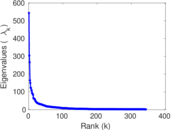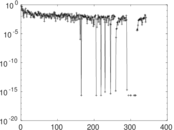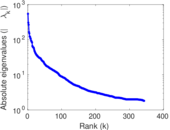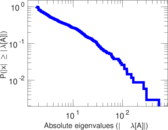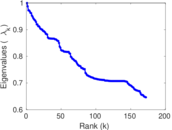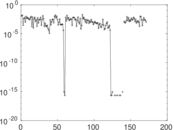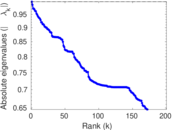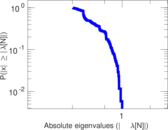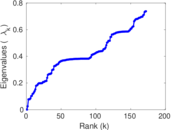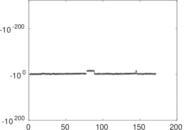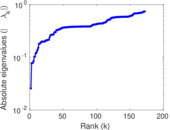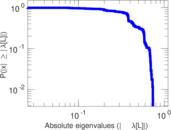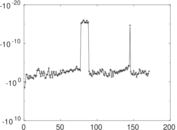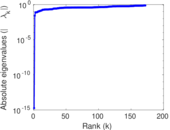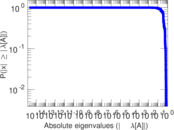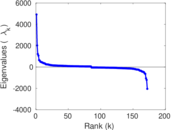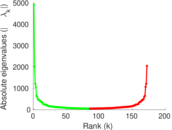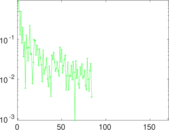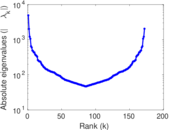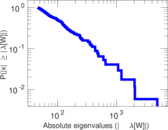# Wikipedia edits (na)

This is the bipartite edit network of the Nauru Wikipedia. It contains users and pages from the Nauru Wikipedia, connected by edit events. Each edge represents an edit. The dataset includes the timestamp of each edit.

 Code `na` Internal name `edit-nawiki` Name Wikipedia edits (na) Data source http://dumps.wikimedia.org/ AvailabilityDataset is available for download Consistency checkDataset passed all tests Category Authorship network Dataset timestamp 2017-10-20 Node meaning User, article Edge meaning Edit Network formatBipartite, undirected Edge typeUnweighted, multiple edges Temporal dataEdges are annotated with timestamps

## Statistics

 Size n = 4,904 Left size n1 = 844 Right size n2 = 4,060 Volume m = 70,695 Unique edge count m̿ = 25,917 Wedge count s = 6,138,057 Claw count z = 1,461,345,694 Cross count x = 331,048,619,422 Square count q = 22,974,300 4-Tour count T4 = 208,415,678 Maximum degree dmax = 6,471 Maximum left degree d1max = 6,471 Maximum right degree d2max = 266 Average degree d = 28.831 6 Average left degree d1 = 83.761 8 Average right degree d2 = 17.412 6 Fill p = 0.007 563 39 Average edge multiplicity m̃ = 2.727 75 Size of LCC N = 4,335 Diameter δ = 12 50-Percentile effective diameter δ0.5 = 3.416 54 90-Percentile effective diameter δ0.9 = 5.483 13 Median distance δM = 4 Mean distance δm = 3.940 35 Gini coefficient G = 0.861 778 Balanced inequality ratio P = 0.144 515 Left balanced inequality ratio P1 = 0.078 591 1 Right balanced inequality ratio P2 = 0.174 878 Relative edge distribution entropy Her = 0.792 654 Power law exponent γ = 2.009 16 Tail power law exponent γt = 1.681 00 Tail power law exponent with p γ3 = 1.681 00 p-value p = 0.000 00 Left tail power law exponent with p γ3,1 = 1.631 00 Left p-value p1 = 0.000 00 Right tail power law exponent with p γ3,2 = 3.691 00 Right p-value p2 = 0.000 00 Degree assortativity ρ = −0.172 567 Degree assortativity p-value pρ = 2.151 46 × 10−172 Spectral norm α = 543.923 Algebraic connectivity a = 0.025 623 4 Spectral separation |λ1[A] / λ2[A]| = 1.787 39 Controllability C = 3,300 Relative controllability Cr = 0.679 292

## Plots

### Fruchterman–Reingold graph drawing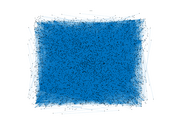### Degree distribution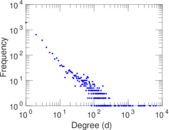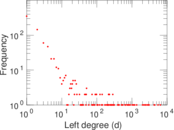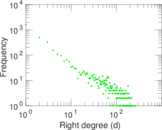### Cumulative degree distribution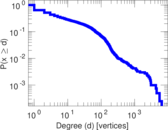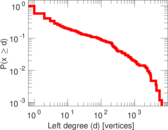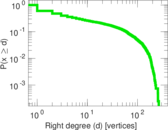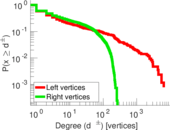### Lorenz curve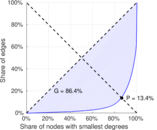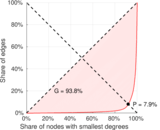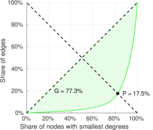### Spectral distribution of the adjacency matrix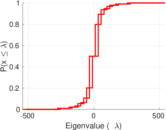### Spectral distribution of the normalized adjacency matrix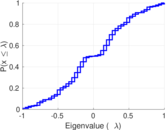### Spectral distribution of the Laplacian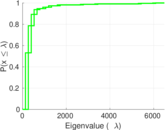### Spectral graph drawing based on the adjacency matrix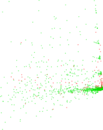### Spectral graph drawing based on the Laplacian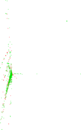### Spectral graph drawing based on the normalized adjacency matrix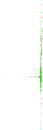### Degree assortativity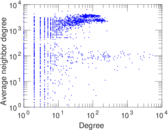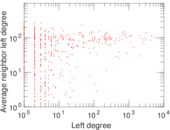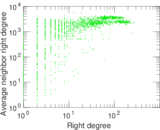### Zipf plot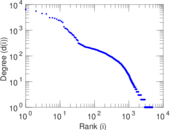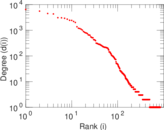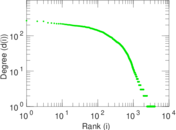### Hop distribution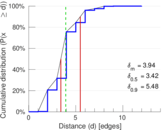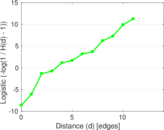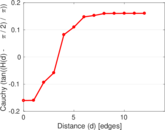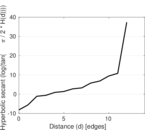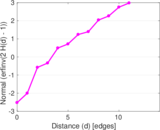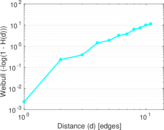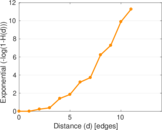### Double Laplacian graph drawing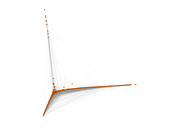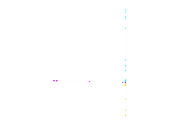### Delaunay graph drawing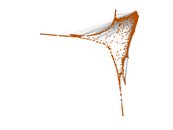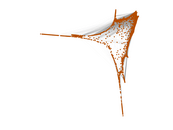### Edge weight/multiplicity distribution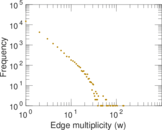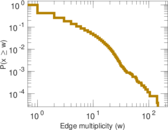### Temporal distribution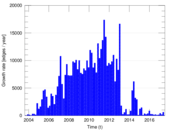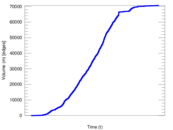### Temporal hop distribution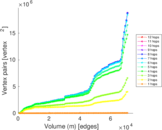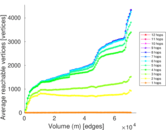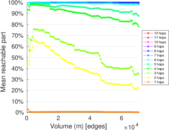### Diameter/density evolution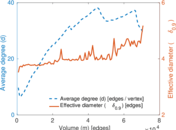### Matrix decompositions plots